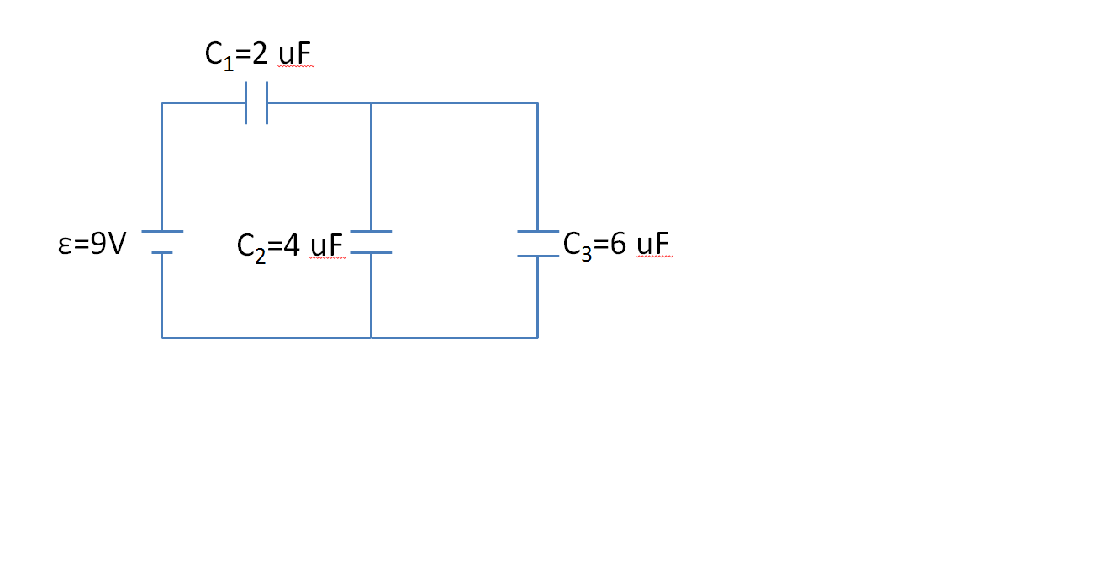# Problem: Three capacitors are connected in the circuit as shown in the figure. (a) What is the equivalent capacitance in the circuit? (b) What is the energy stored in each capacitor?

###### FREE Expert Solution
90% (397 ratings)
###### Problem Details

Three capacitors are connected in the circuit as shown in the figure.

(a) What is the equivalent capacitance in the circuit?

(b) What is the energy stored in each capacitor?